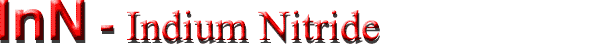## Mobility and Hall Effect

 Wurtzite InN Remarks Referens Conductivity σ 2 ÷3 Ω-1 cm-1 300 K ; temperature coefficient of resistivity 3.7·10-3 K-1 at 200...300K for pressed powder Juza et al. (1956); Hovel & Cuomo (1972); Trainor & Rose (1974) Mobility electrons μn 250(50) cm2 V-1 s-1 300 K ; see also Electron mobility vs. temperature Hovel & Cuomo (1972) 20 cm2 V-1 s-1 Trainor & Rose (1974) 35 ... 50 cm2 V-1 s-1 Marasina et al. (1977)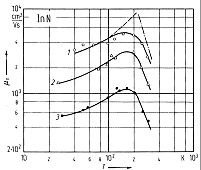InN, Wurtzite. Electron Hall mobility vs. temperature for three samples with RT and carrier concentrations: (1) - 5.3x1016 cm-3; (2) - 7.5x1016 cm-3; (3) - 1.8x1017 cm-3. Broken line: calculated ionized impurity scattering mobility, Dot-dashed line: empirical high-temperature mobility (μ~T-3) for sample (1) Solid lines: total mobility calculated for each sample. Tansley & Tansley (1984)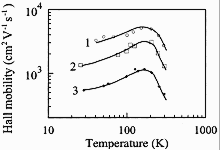InN, Wurtzite, polycrystalline films. Electron Hall mobility versus temperature for different doping levels and different degrees of compensation θ = Na/Nd. 1 - Nd = 5.1 x 1016 cm-3, θ ~= 0.3; 2 - Nd = 8.7 x 1016 cm-3, θ ~= 0.6; 3 - Nd Ā= 3.9 x 1017 cm-3, θ ~=0.8 Tansley & Tansley (1984).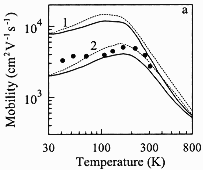InN, Wurtzite sructure. Electron drift (solid curves) and Hall (dashed curves) mobility versus temperature calculated for carrier concentration at different compensation ratios θ: n0 = 5 x 1016 cm-3; 1 - θ = 0;     2 - θ = 0.60;     Experimental data are taken from Tansley & Foley (1984) Chin et al. (1994).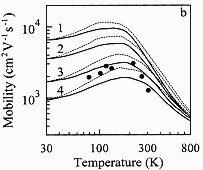InN, Wurtzite sructure. Electron drift (solid curves) and Hall (dashed curves) mobility versus temperature calculated for carrier concentration at different compensation ratios θ: n0 = 8x 1016 cm-3; 1 - θ = 0;     2 - θ = 0.30; 3 - θ = 0.60;     4 - θ = 0.75; Experimental data are taken from Tansley & Foley (1984) Chin et al. (1994).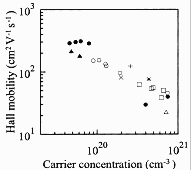InN, Wurtzite sructure. The electron Hall mobility versus carrier concentration. InN films grown on sapphire and GaAs substrates Yamamoto et al. (1998)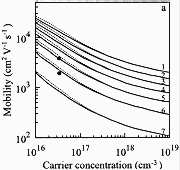InN, Wurtzite sructure. The calculated electron drift (solid curves) and Hall (dashed curves) mobility versus carrier concentration at different compensation ratios θ: T = 77K. 1 - θ = 0;          2 - θ = 0.15;     3 - θ = 0.30;     4 - θ = 0.45; 5 - θ = 0.60;     6 - θ = 0.75;     7 - θ = 0.90. Experimental data are taken from Tansley & Foley (1984) Chin et al. (1994).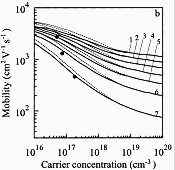InN, Wurtzite sructure. The calculated electron drift (solid curves) and Hall (dashed curves) mobility versus carrier concentration at different compensation ratios θ: T = 300K. 1 - θ = 0;          2 - θ = 0.15; 3 - θ = 0.30;     4 - θ = 0.45; 5 - θ = 0.60;     6 - θ = 0.75;     7 - θ = 0.90. Experimental data are taken from Tansley & Foley (1984) Chin et al. (1994).